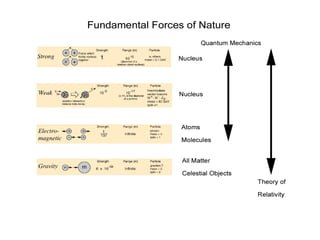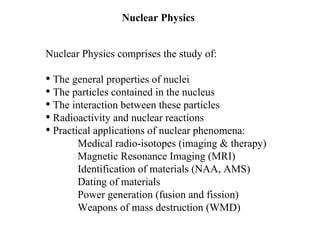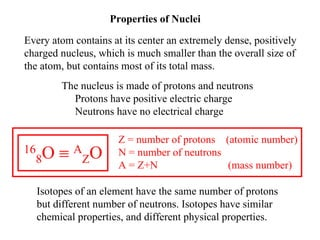Se está descargando tu SlideShare. ×

# Norman John Brodeur Nuclear Physics.pdf

Anuncio
Anuncio
Anuncio
Anuncio
Anuncio
Anuncio
Anuncio
Anuncio
Anuncio
Anuncio
AnuncioCargando en…3
×

1 de 28 Anuncio

# Norman John Brodeur Nuclear Physics.pdf

Norman John Brodeur worked at MIT’s instrumentation lab which later became Draper Labs. My responsibility was instrumentation and guidance systems for the Apollo command module and the lunar module. Previous to that I worked for Avco-Everett Research Lab in Everett. There we focused on testing materials for the vehicle’s heat shield. I was doing heat studies of various materials and what we eventually developed would just burn off and the heat with it.

Norman John Brodeur worked at MIT’s instrumentation lab which later became Draper Labs. My responsibility was instrumentation and guidance systems for the Apollo command module and the lunar module. Previous to that I worked for Avco-Everett Research Lab in Everett. There we focused on testing materials for the vehicle’s heat shield. I was doing heat studies of various materials and what we eventually developed would just burn off and the heat with it.

Anuncio
Anuncio

### Norman John Brodeur Nuclear Physics.pdf

1. 1. Nuclear Physics Nuclear Physics comprises the study of: • The general properties of nuclei • The particles contained in the nucleus • The interaction between these particles • Radioactivity and nuclear reactions • Practical applications of nuclear phenomena: Medical radio-isotopes (imaging & therapy) Magnetic Resonance Imaging (MRI) Identification of materials (NAA, AMS) Dating of materials Power generation (fusion and fission) Weapons of mass destruction (WMD)
2. 2. Properties of Nuclei Every atom contains at its center an extremely dense, positively charged nucleus, which is much smaller than the overall size of the atom, but contains most of its total mass. The nucleus is made of protons and neutrons Protons have positive electric charge Neutrons have no electrical charge Isotopes of an element have the same number of protons but different number of neutrons. Isotopes have similar chemical properties, and different physical properties. Z = number of protons (atomic number) N = number of neutrons A = Z+N (mass number) 16 8O ≡ A ZO
3. 3. Isotope A Z N Atomic Mass (u) Abundance 12 6C 12 6 6 12.000000 98.90% 13 6C 13 6 7 13.003355 1.10% [The chemical atomic weight of carbon is 12.011 u] 14 6C 14 6 8 14.003242 Radioactive [14 6C decays by β- emission with Half-Life 5730 y]
4. 4. Nuclear Size and Mass The radii of most nuclei is given by the equation: R = R0 A1/3 R: radius of the nucleus, A: mass number, R0 = 1.2x10-15 m The mass of a nucleus is given by: m = A u u = 1.66…x10-27 kg (unified mass unit)
5. 5. Nuclear Density 56 26Fe is the most abundant isotope of iron (91.7%) R = 1.2x10-15 m (56)1/3 = 4.6x10-15 m V = 4/3 π R3 = 4.1x10-43 m3 m = (56) 1.66x10-27 kg = 9.3x10-26 kg ρ = m / V = 2.3x1017 kg/m3 [Compare with the density of solid iron ≈ 7x103 kg/m3] Since: m = A u and R = R0 A1/3 ρ = m / V = u / (4/3 π R0 3) ≈ constant All nuclei have approximately the same density [Compare with inter-atomic separations ≈ 0.1x10-9 – 0.3x10-9 m]
6. 6. Nuclear Binding Energy The total rest energy of the separated nucleons is greater than the rest energy of the nucleus. The difference is called the Binding Energy EB EB is a measure of the energy gained in forming the nucleus from the individual nucleons Note that A ZM is the mass of the neutral atom containing the nucleus, and MH refers to the mass of hydrogen (to balance the Z electrons contained in A ZM) EB = (Z MH + N mN – A ZM) c2
7. 7. Binding Energy per Nucleon The binding energy per nucleon EB/A is nearly constant as a function of A, suggesting that the nuclear force is saturated (a nucleon interacts only with its nearest neighbors).
8. 8. The Nuclear Force The force that binds together protons and neutrons inside the nucleus is called the Nuclear Force Some characteristics of the nuclear force are: • It does not depend on charge • It is very short range ≈ 10-15 m • It is much stronger than the electric force • It is saturated (nucleons interact only with near neighbors) • It favors formation of pairs of nucleons with opposite spins We do not have a simple equation to describe the nuclear force
9. 9. Nuclear Stability The energy of a group of nucleons in a square well ( a reasonable first approximation to a nucleus) is smaller if Z ≅ N. For large values of A we need N ≥ Z to compensate for the electric force
10. 10. Nuclear Stability Plot of N vs. Z for known nuclides. The stable nuclides are indicated by the black dots. Non-stable nuclides decay by emission of particles, or electromagnetic radiation, in a process called radioactivity
11. 11. Radioactivity Alpha Decay: nuclei that are too large to be stable tend to decay by alpha decay, the emission of an alpha particle. [An alpha particle is the 4He nucleus, two protons and two neutrons] 238 92U → 234 90Th + α Gamma Decay: the energy of internal motion (protons and neutrons in a nucleus is quantized. A nucleus has a set of allowed energy states (ground state and excited states) much like in an atom. Transitions between states lead to the emission of very energetic electromagnetic radiation called γ (gamma) rays 238 92U* → 238 92U + γ
12. 12. Radioactivity Beta Decay: consist in a) the emission of an electron β- , b) the emission of a positron β+, or c) electron capture. a) Nuclides with neutron to proton ratio too large for stability tend to decay by conversion of a neutron into a proton, an electron and an antineutrino: n → p + β- + ν b) Nuclides with proton to neutron ratio too large for stability tend to decay by conversion of a proton into a neutron, a positron, and a neutrino: p → n + β+ + ν c) In certain cases an orbital electron can combine with a proton in the nucleus to form a neutron and a neutrino: p + β- → n + ν
13. 13. Radioactivity Radioactive decay is a statistical process. There is now way to predict when an individual nucleus will decay. However, it is possible to predict the decay rate of a group of nuclei. The constant λ is called the decay constant and can be interpreted as the probability per unit time that a nucleus will decay If the initial number of radioactive nuclei at time t = 0 is N0, then the number of remaining nuclei at time t is given by: N(t) = N0 exp(-λt)
14. 14. Radioactivity The Half-Life T1/2 is the time required for the number of radioactive nuclei to decrease to half the initial value N0 N0/2 = N0 exp(-λT1/2) ⇒ T1/2 = ln 2 / λ The mean lifetime Tmean, or lifetime, is the time required for the number of radioactive nuclei to decrease to 1/e of the initial value N0 λTmean = 1 ⇒ Tmean = 1 / λ The number of decays per unit time dN/dt is the activity of a sample, and is measured in Curie (Ci) ⇒ 1 Ci = 3.7x1010 decays/second t1/2 ≡ T1/2 τ ≡ Tmean
15. 15. 238U decay series
16. 16. 14C Dating Radioactive 14C is continuously produced in the atmosphere [14N(n,p)14C, the neutrons being produced by cosmic rays]. 14C decays by β- emission with half life T 1/2 = 5730 y 14C → 14N + β- + ν The chemical activity of 14C is similar to that of 12C, so living organisms have the same 14C /12C ratio as in the atmosphere, which is about 1.35x10-12. When an organism dies it stops absorbing 14C, and the ratio 14C /12C decreases. Measurement of this ratio allows the calculation of the time of death of the organism.
17. 17. Nuclear Reactions A nuclear reaction is a rearrangement of nuclear components induced by particle bombardment 4 2He + 14 7N → 17 8O + 1 1H 14 7N(α,p)17 8O Nuclear reactions are subject to the following conservation laws: Charge Momentum and angular momentum Energy Total number of nucleons
18. 18. Nuclear Reactions Some nuclear reactions release energy, while other reactions require input energy to proceed The amount of energy released or absorbed in a nuclear reaction (in the center of mass reference frame) is called the Q value, or reaction energy: If MA + MB > MC + MD → Q > 0 ≡ exoergic reaction If MA + MB < MC + MD → Q < 0 ≡ endoergic reaction An endoergic reaction will not proceed unless the incoming particle provides the reaction energy Q (in CM) In a reaction: MA + MB → MC + MD Q = (MA + MB - MC - MD) c2
19. 19. Particle Induced Nuclear Reactions: are used mostly for nuclear physics research, and for analytical purposes The reaction 7Li(p,α)4He can be used to detect Li in solids [1-2 MeV protons]
20. 20. Neutron Induced Nuclear Reactions Nuclear reactions induced by neutron bombardment are used: a) In analytical techniques such as neutron activation analysis b) In the generation of energy by Fission or Fusion It is convenient to distinguish between fast and slow neutrons Fast neutron → kinetic energy ≥ 1 MeV Slow neutron or thermal neutron → kinetic energy ~ 0.025 eV The probability for a reaction to proceed or Cross Section depends strongly on the energy of the neutrons
21. 21. Neutron Activation Analysis Various layers of a painting are revealed using NAA Low energy neutrons are likely to be captured by nuclei, with emission of radiation from the excited nucleus, as in X(n,γ)Y. The emitted radiation is a signature for the presence of element X
22. 22. Fission and Fusion The binding energy per nucleon peaks for A ~ 70, then: a) splitting a large mass nucleus into two medium mass nuclei (fission), or b) fusing two low mass nuclei into a larger mass nucleus (fusion) result in the release of energy. Binding energy per nucleon
23. 23. Fission Uranium has two main isotopes: 238U with an abundance of 99.3% 235U with an abundance of 0.7% When 235U captures a thermal, or low energy, neutron it forms 236U that decays by undergoing fission (85% of the time) n + 235U → 141Ba + 92Kr + 3n There is about 1 MeV per nucleon higher binding energy in the products of the reaction than in the Uranium. As a consequence, more that 200 MeV of energy are liberated in this reaction. Furthermore, the emitted neutrons can induce additional fission
24. 24. Chain Fission Reaction To sustain a chain reaction one of the emitted neutrons must be captured by another 235U nucleus The reproduction constant k is the average number of neutrons from each fission that cause subsequent fission k < 1 → the reaction will die out k ≈ 1 → the reaction will be sustained (nuclear reactor) k > 1 → the reaction will run away (nuclear bomb)
25. 25. Nuclear Fission Reactor Fission of 235U is most likely for low energy neutrons. The neutrons emitted in fission are more energetic so they need to be slowed down by means of a moderator (water, graphite) placed in between the fuel rods. Control rods, of Cadmium or other materials, are further used to regulate the number of fission inducing neutrons
26. 26. Fusion In fusion, two light nuclei such as deuterium 2H and tritium 3H fuse together to form a heavier nucleus: 2H + 3H → 4He + n + 17.6 MeV The energy released per nucleon is larger than that released in fission The production of power from fusion of light nuclei holds great promise because of the relative abundance of fuel, and the absence of some of the dangers inherent in fission. However, the technology necessary to make fusion practical, has not been developed yet.
27. 27. Fusion The proton – proton cycle, resulting in the formation of 4He, and the release of considerable energy, is the primary source of the sun’s energy In practice it is very difficult to generate the necessary density of super-hot gas (T > 107 K - plasma), and keep it confined, to facilitate the fusion process.